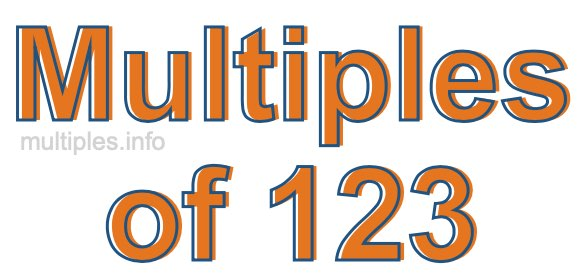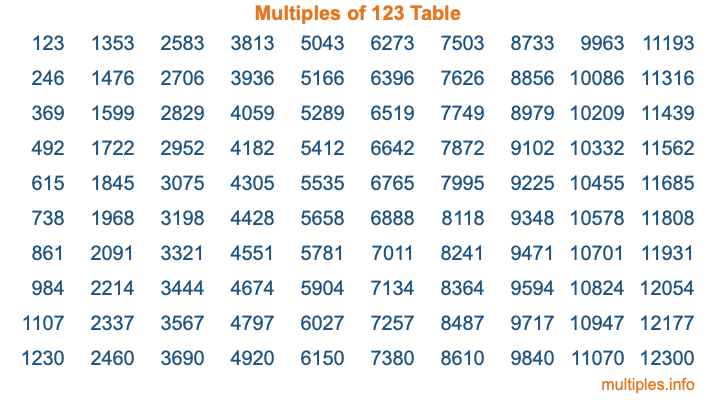Multiples of 123Welcome to the Multiples of 123 page. Here we will first teach you everything you will ever need to know about the multiples of 123, and then give you a study guide summary of everything we taught you to make sure you remember it all. Use this page to look up facts and learn information about the multiples of 123. This page will make you a multiples of one hundred twenty-three expert!

Definition of Multiples of 123
Multiples of 123 are all the numbers that when divided by 123 equal an integer. Each of the multiples of 123 are called a multiple. A multiple of 123 is created by multiplying 123 by an integer.

Therefore, to create a list of multiples of 123, you start with 1 multiplied by 123, then 2 multiplied by 123, then 3 multiplied by 123, and so on for as long as you want. Thus, the list of the first five multiples of 123 is 123, 246, 369, 492, and 615. To see a larger list of multiples of 123, see the printable image of Multiples of 123 further down on this page. We also have a category where you can choose any nth multiple of 123.

Multiples of 123 Checker
The Multiples of 123 Checker below checks to see if any number of your choice is a multiple of 123. In other words, it checks to see if there is any number (integer) that when multiplied by 123 will equal your number. To do that, we divide your number by 123. If the the quotient is an integer, then your number is a multiple of 123.

Is  a multiple of 123?

Least Common Multiple of 123 and ...
A Least Common Multiple (LCM) is the lowest multiple that two or more numbers have in common. This is also called the smallest common multiple or lowest common multiple and is useful to know when you are adding our subtracting fractions. Enter one or more numbers below (123 is already entered) to find the LCM.

Check out our LCM Calculator if you need more details about the Least Common Multiple or if you need the LCM for different numbers for adding and subtraction fractions.

nth Multiple of 123
As we stated above, 123 is the first multiple of 123, 246 is the second multiple of 123, 369 is the third multiple of 123, and so on. Enter a number below to find the nth multiple of 123.

th multiple of 123

Multiples of 123 vs Factors of 123
123 is a multiple of 123 and a factor of 123, but that is where the similarities end. All postive multiples of 123 are 123 or greater than 123. All positive factors of 123 are 123 or less than 123.

Below is the beginning list of multiples of 123 and the factors of 123 so you can compare:

Multiples of 123: 123, 246, 369, 492, 615, etc.

Factors of 123: 1, 3, 41, 123

As you can see, the multiples of 123 are all the numbers that you can divide by 123 to get a whole number. The factors of 123, on the other hand, are all the whole numbers that you can multiply by another whole number to get 123.

It's also interesting to note that if a number (x) is a factor of 123, then 123 will also be a multiple of that number (x).

Multiples of 123 vs Divisors of 123
The divisors of 123 are all the integers that 123 can be divided by evenly. Below is a list of the divisors of 123.

Divisors of 123: 1, 3, 41, 123

The interesting thing to note here is that if you take any multiple of 123 and divide it by a divisor of 123, you will see that the quotient is an integer.

Multiples of 123 Table
Below is an image of the first 100 multiples of 123 in a table. The table is in chronological order, column by column. The first column has the first ten multiples of 123, the second column has the next ten multiples of 123, and so on.The Multiples of 123 Table is also referred to as the 123 Times Table or Times Table of 123. You are welcome to print out our table for your studies.

Negative Multiples of 123
Although not often discussed or needed in math, it is worth mentioning that you can make a list of negative multiples of 123 by multiplying 123 by -1, then by -2, then by -3, and so on, to get the following list of negative multiples of 123:

-123, -246, -369, -492, -615, etc.

Multiples of 123 Summary
Below is a summary of important Multiples of 123 facts that we have discussed on this page. To retain the knowledge on this page, we recommend that you read through the summary and explain to yourself or a study partner why they hold true.

There are an infinite number of multiples of 123.

A multiple of 123 divided by 123 will equal a whole number.

123 divided by a factor of 123 equals a divisor of 123.

The nth multiple of 123 is n times 123.

The largest factor of 123 is equal to the first positive multiple of 123.

123 is a multiple of every factor of 123.

123 is a multiple of 123.

A multiple of 123 divided by a divisor of 123 equals an integer.

123 divided by a divisor of 123 equals a factor of 123.

Any integer times 123 will equal a multiple of 123.

Multiples of a Number
Here you can get the multiples of another number, all with the same attention to detail as we did for multiples of 123 on this page.

Multiples of
Multiples of 124
Did you find our page about multiples of one hundred twenty-three educational? Do you want more knowledge? Check out the multiples of the next number on our list!